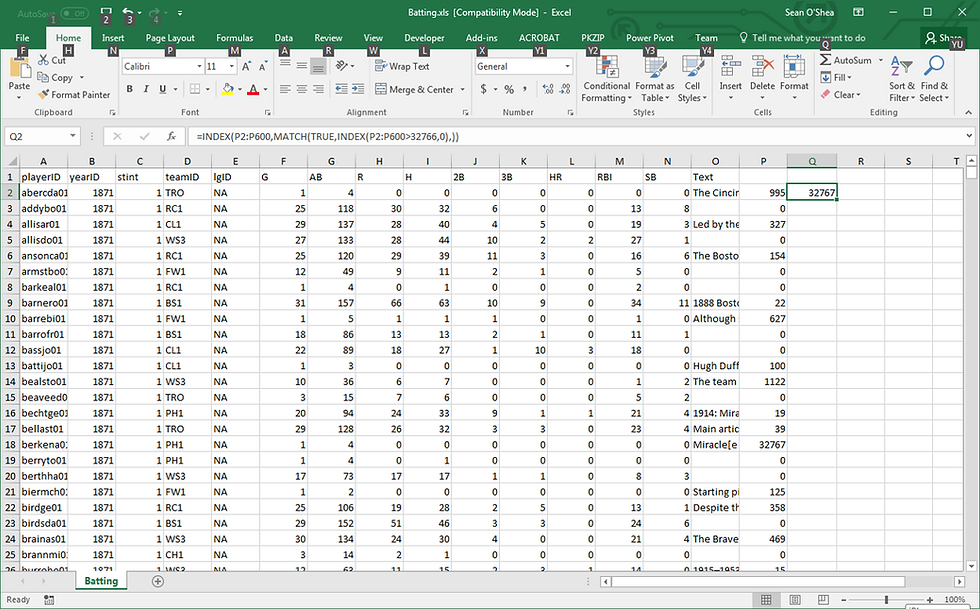top of pageSearch

# INDEX MATCH formula to find value greater than X

You can use this INDEX MATCH formula to find the first value in a column greater than the specified value.

=INDEX(A2:A16,MATCH(TRUE,INDEX(A2:A16>150,0),))

I like to use this to check and see if any cell in a worksheet has a value of 32767, the upper character limit for a single cell in Excel. In this example I have run a LEN formula in column P to check the number of characters in each cell in column O. The INDEX MATCH formula is entered in column Q to check and see if there any instances of a cell that has reached the maximum - a sign that at some point in the processing of the data the text may have been cut off.

=INDEX(P2:P600,MATCH(TRUE,INDEX(P2:P600>32766,0),))bottom of page